# How To Using Sheet Names In Formulas Excel

Feb 01, 2020 • edited Feb 02, 2020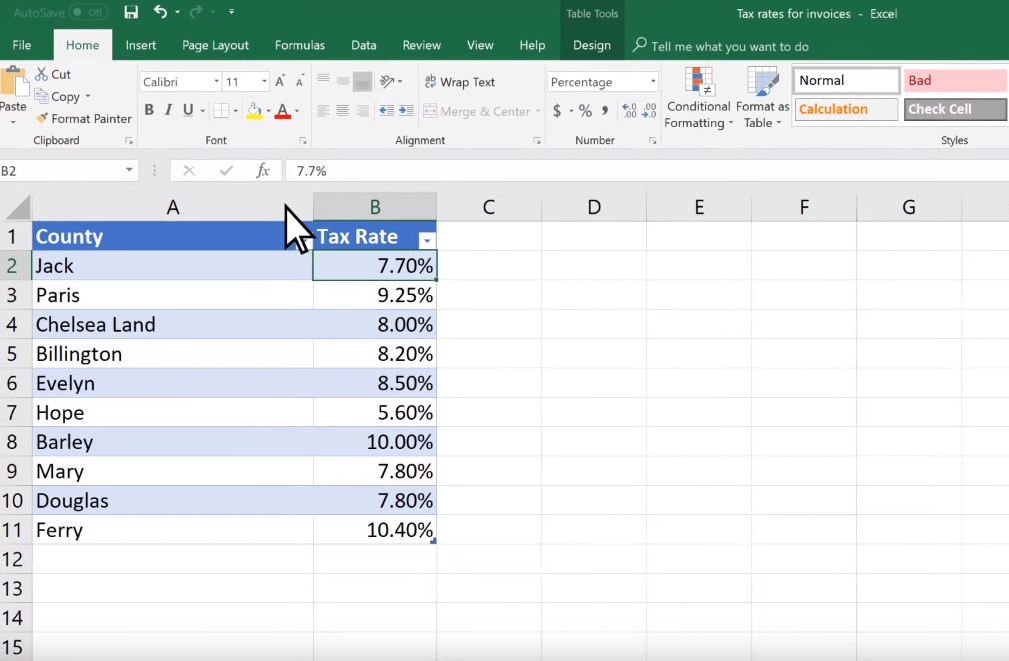For referencing the current sheet tab name in a cell in Excel, you can get it done with a formula or User Define Function.

In Excel, you can name cells and cell ranges, and use those names in formulas. This is a useful feature that makes formulas easier to understand and maintain.

Create a named range or a named constant and use these names in your formulas. This way you can make your formulas easier to understand.

### Named Range

To create a named range, execute the following steps.

1. Select the range A1:A4.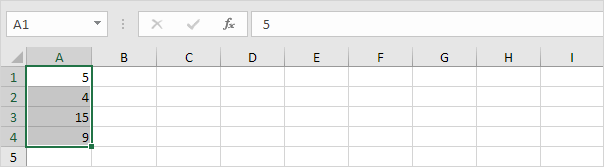2. On the Formulas tab, in the Defined Names group, click Define Name.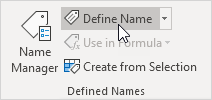3. Enter a name and click OK.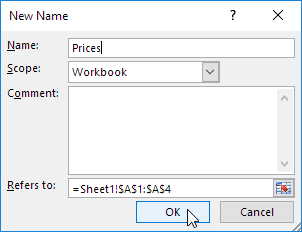There's an even quicker way of doing this.

4. Select the range, type the name in the Name box and press Enter.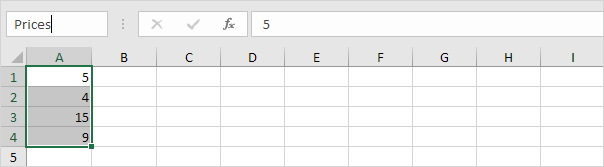5. Now you can use this named range in your formulas. For example, sum Prices.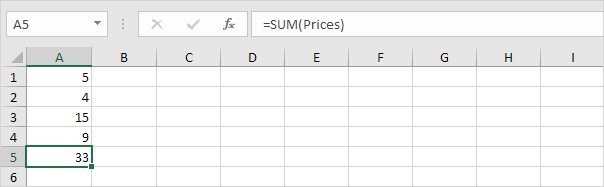### Named Constant

To create a named constant, execute the following steps.

1. On the Formulas tab, in the Defined Names group, click Define Name.2. Enter a name, type a value, and click OK.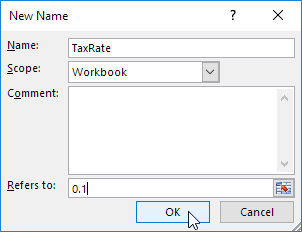3. Now you can use this named constant in your formulas.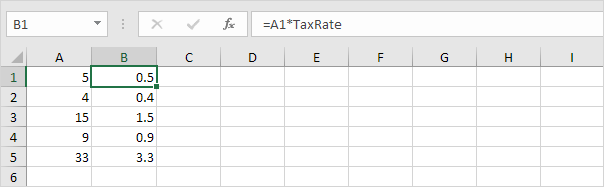Note: if the tax rate changes, use the Name Manager to edit the name and Excel automatically updates all the formulas that use TaxRate.

### Name Manager

To edit and delete defined names, execute the following steps.

1. On the Formulas tab, in the Defined Names group, click Name Manager.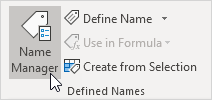2. For example, select TaxRate and click Edit.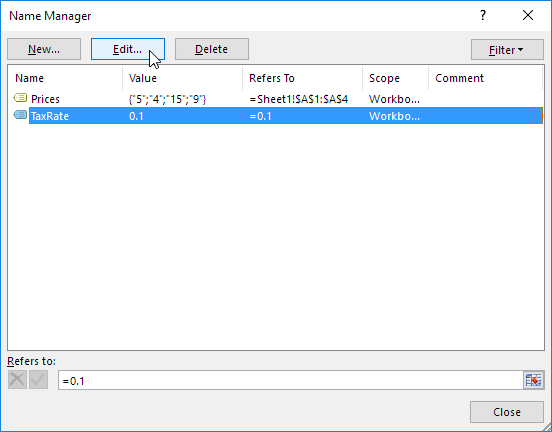#How To#Tutorial#Formulas#Functions

The various paste options in Excel

How to Calculating percentages with Formulas in Excel